Courses

# Previous Year Questions - Areas Related to Circles (part-1) Class 10 Notes | EduRev

## Class 10 : Previous Year Questions - Areas Related to Circles (part-1) Class 10 Notes | EduRev

The document Previous Year Questions - Areas Related to Circles (part-1) Class 10 Notes | EduRev is a part of the Class 10 Course Mathematics (Maths) Class 10.
All you need of Class 10 at this link: Class 10

Short Answer Type Questions

Q.1. Find the area of the shaded region in Fig., if ABCD is a rectangle with sides 8 cm and 6 cm and O is the centre of circle. [Take π = 3.14]      [Delhi 2019]
Ans.
O is the centre of the circle.
AC is the diameter.
∴ In ΔADC, ∠D = 90°
∴ AC2=AD2+CD2
AC2=(6)2+(8)2 ⇒ AC2 = 36+64
⇒ AC2=100 ⇒AC=10 cm
OC = radius of circle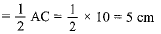Area of the circle = πr2 = 3.14(5)2
= 25 x 3.14 = 78.5 cm2
Area of the rectangle = 6 x 8 = 48 cm2
∴ Area of the shaded region = area of the circle - area of the rectangle
= 78.5 - 48 = 30.5 cm2

Q.2. A chord of a circle of radius 12 cm subtends an angle of 120° at the centre. Find the area of the corresponding segment of the circle (Use π = 3.14 and √3 = 1.73)     [Delhi 2019]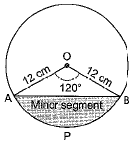Ans.
We have, r = 12 cm and θ = 120°
Now, area of the corresponding segment APB of circle
= area of the minor segment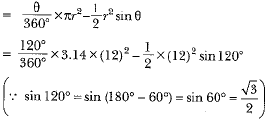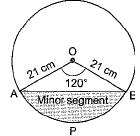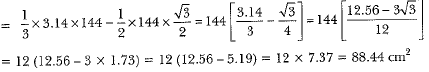Q.3. In the figure, three section of a circle of radius 7 cm, making angles of 60°, 80° and 40° at the centre are shaded. Find the area of the shaded region.    [CBSE 2019 (30/5/1)]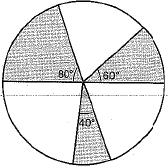Ans.
We have radius of circle = 7 cm
Area of sector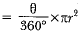Area of sector containing 60°angle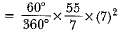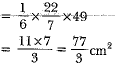Also, area of sector containing 80° angle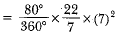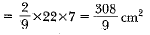Again, area of sector containing 40° angle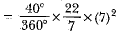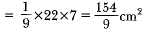Area of total shaded region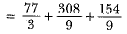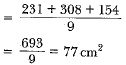Q.4. In figure, a square OABC is inscribed in a quadrant OPBQ. If OA = 15 cm, find the area of the shaded region. (Use π = 3.14)     [Allahabad 2019]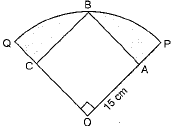Ans.
OB2 =OA2 + AB2
OB2 = (15)2 + (15)2
OB2 =225 + 225
OB2 =450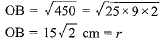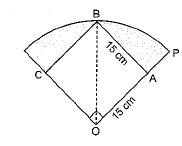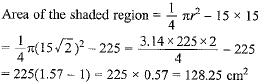Q.5. Find the area of the shaded region in Fig., where arcs drawn with centres A, B, C and D intersect in pairs at mid-points P, Q, R and S of the sides AB, BC, CD and DA respectively of a square ABCD of side 12 cm. [Use π - 3.14]     [CBSE 2018]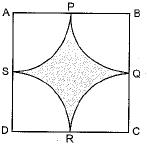Ans.
Radius of each quadrant = 12/2 = 6 cm
Area o f each quadrant =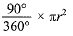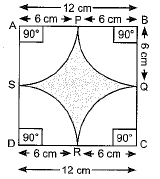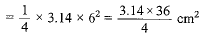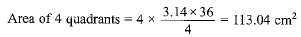Area of square = (side)2 = (12)2 = 144 cm2
Area of the shaded region = ar(square) - ar(4 quadrants)
= 144 cm2 - 113.04 cm2 = 30.96 cm2

Q.6. The side of a square is 10 cm. Find the area between inscribed and circumscribed circles of the square.    [CBSE 2018 (C)]
Ans.
ABCD is a square. A circle with centre O and radius r is inscribed in the square.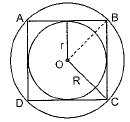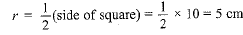OP = r = 5 cm
In ΔOPB
OB2 = OP2 + PB2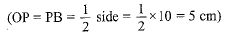= 52 + 52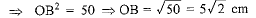Area of circumscribed circle with radius (R) = πR2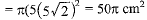Area of inscribed circle with radius (r)
= πr2 = π(5)2 = 25π cm2
Area between inscribed and circumscribed circles of square is shaded area with dots and lines.
= (50π - 25π) cm2 = 25π cm2

Q.7. The short and long hands of a clock are 4 cm and 6 cm long respectively. Find the sum of distances travelled by their tips in 48 hours.     [CBSE 2018 (C)]
Ans.
The length of short hand of the clock = 4 cm
Short hand covers one complete path in 12 hours Path traced by short hand in 12 hour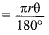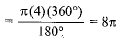Path traced by short hand in 48 hours = 4 x 8π = 32π
Long hand covers one complete path in 1 hour
Path traced by long hand in 1 hour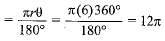Path traced by long hand in 48 hours
= 48 x 12π = 576π
Sum of distances travelled by their tips in 48 hours
= 32π + 576π = 608π

Q.8. Three semicircle each of diameter 3 cm, a circle of diameter 4.5 cm and a semicircle of radius 4.5 cm are drawn in the given figure. Find the area of the shaded region.     [AI 2017]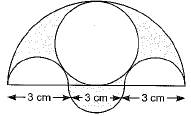Ans.
In figure
Areas A, B and C are identical semicircles each having diameter 3 cm.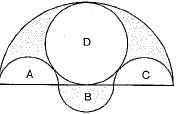So, radius of each of these semicircle = 3/2 cm
Area E is a semicircle with diameter
= 3 + 3 +3 = 9 cm
∴ Radius of area E= 9/2 cm
Area D is a complete circle with diameter 4.5 cm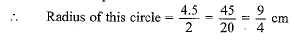The required shaded area = E + B - A - C - D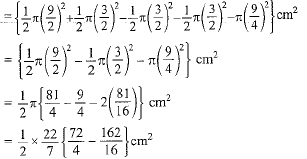Hence, the required shaded area is 12.38 cm2

Q.9. The difference between the radii of the smaller circle and the larger circle is 7 cm and the difference between the areas of the two circles is 1078 sq. cm. Find the radius of the smaller circle.    [Delhi 2017 C]
Ans.
Let radius of smaller circle be r cm
radius of larger circle be R cm
also, R - r = 7 cm ,     ..(i)
difference between the areas of the two circles
πR2 - πr2
Here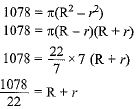R + r = 49 cm    ...(ii)
solving (i) and (ii) for R and r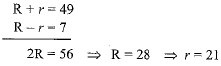Q.10.  In Fig., ABCD is a trapezium with AB||DC and ∠BCD = 30°. If BGEC is a sector of a circle with centre C and AB = BC = 7 cm, DE = 4 cm and BF = 3.5 cm, then find the area of the shaded region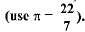[AI 2017 C]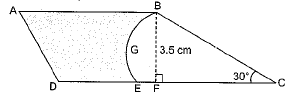Ans.
BC = 7 cm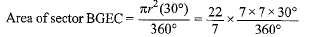= 12.83 cm2
CD = DE + EC = 4 + 7 = 11 cm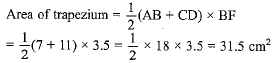Area of shaded region = Area of trapezium - Area of sector BGEC
= (31.5)-(12.83) = 18.67 cm2

Q.11. A wire when bent in the form of an equilateral triangle encloses an area of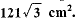If the wire is bent in the form of a circle, find the area enclosed by the circle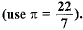[AI 2017 (C)]
Ans.
Let side of the equilateral triangle is a cm
∴ Area o f the equilateral triangle =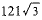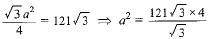a2 = (121)4
a = 11 x 2 = 22 cm
The wire is bent in the form of circle having radius r cm
∴ Circumference of the circle = perimeter of the equilateral Δ
∴ 2πr = 3 x 22r = 10.5 cm
Area of circle = πr2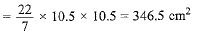Q.12. In Fig. 12.27, OACB is a quadrant of a circle with centre O and radius 3.5 cm. If OD = 2 cm, find the area of the (i) quadrant OACB, (ii) shaded region.      [CBSE Delhi 2017]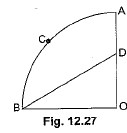Ans.
(i) Area o f quadrant OACB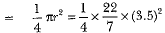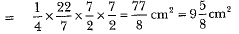(ii) Now, we find area of ΔOBD
We have area of ΔOBD =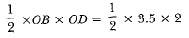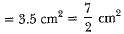Hence, area of shaded region = area o f quadrant OACB - area of ΔOBD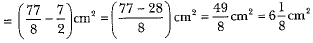Offer running on EduRev: Apply code STAYHOME200 to get INR 200 off on our premium plan EduRev Infinity!

## Mathematics (Maths) Class 10

62 videos|363 docs|103 tests

,

,

,

,

,

,

,

,

,

,

,

,

,

,

,

,

,

,

,

,

,

;